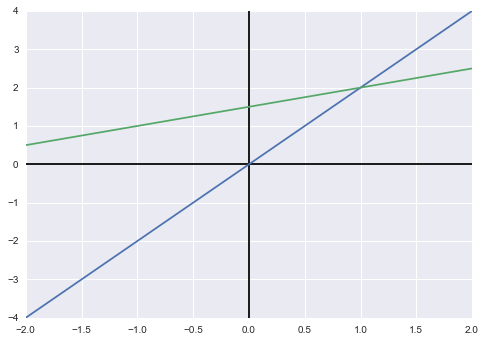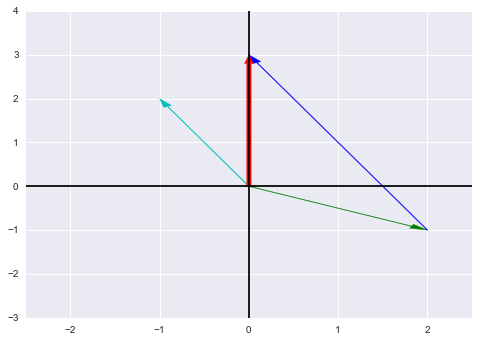# 第一讲：方程组的几何解释

%matplotlib inline
import matplotlib.pyplot as plt
import numpy as np
import pandas as pd
import seaborn as sns

x = [-2, 2, -2, 2]
y = [-4, 4, 0.5, 2.5]

fig = plt.figure()
plt.axhline(y=0, c='black')
plt.axvline(x=0, c='black')

plt.plot(x[:2], y[:2], x[2:], y[2:])

plt.draw()plt.close(fig)


from functools import partial

fig = plt.figure()
plt.axhline(y=0, c='black')
plt.axvline(x=0, c='black')
ax = plt.gca()
ax.set_xlim(-2.5, 2.5)
ax.set_ylim(-3, 4)

arrow_vector(0, 0, 2, -1, color='g')
arrow_vector(0, 0, -1, 2, color='c')
arrow_vector(2, -1, -2, 4, color='b')
arrow_vector(0, 0, 0, 3, width=0.05, color='r')

plt.draw()plt.close(fig)


——如果三个向量在同一个平面上，问题就出现了——那么他们的线性组合也一定都在这个平面上。举个例子，比如$$col_3=col_1+col_2$$，那么不管怎么组合，这三个向量的结果都逃不出这个平面，因此当$$b$$在平面内，方程组有解，而当$$b$$不在平面内，这三个列向量就无法构造出$$b$$。在后面的课程中，我们会了解到这种情形称为奇异矩阵不可逆

• 我们依然使用列向量线性组合的方式，一次计算一列，$$\begin{bmatrix}2&5\\1&3\end{bmatrix}\begin{bmatrix}1\\2\end{bmatrix}=1\begin{bmatrix}2\\1\end{bmatrix}+2\begin{bmatrix}5\\3\end{bmatrix}=\begin{bmatrix}12\\7\end{bmatrix}$$
• 另一种方法，使用向量内积，矩阵第一行向量点乘$$x$$向量$$\begin{bmatrix}2&5\end{bmatrix}\cdot\begin{bmatrix}1&2\end{bmatrix}^T=12,\ \begin{bmatrix}1&3\end{bmatrix}\cdot\begin{bmatrix}1&2\end{bmatrix}^T=7$$